# Learn About Independent Events With Example Problems And Interactive Exercises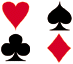Experiment 1: A dresser drawer contains one pair of socks with each of the following colors: blue, brown, red, white and black. Each pair is folded together in a matching set. You reach into the sock drawer and choose a pair of socks without looking. You replace this pair and then choose another pair of socks. What is the probability that you will choose the red pair of socks both times?

There are a couple of things to note about this experiment. Choosing a pairs of socks from the drawer, replacing it, and then choosing a pair again from the same drawer is a compound event. Since the first pair was replaced, choosing a red pair on the first try has no effect on the probability of choosing a red pair on the second try. Therefore, these events are independent.

Definition: Two events, A and B, are independent if the fact that A occurs does not affect the probability of B occurring.

Some other examples of independent events are:

• Landing on heads after tossing a coin AND rolling a 5 on a single 6-sided die.
• Choosing a marble from a jar AND landing on heads after tossing a coin.
• Choosing a 3 from a deck of cards, replacing it, AND then choosing an ace as the second card.
• Rolling a 4 on a single 6-sided die, AND then rolling a 1 on a second roll of the die.

To find the probability of two independent events that occur in sequence, find the probability of each event occurring separately, and then multiply the probabilities. This multiplication rule is defined symbolically below. Note that multiplication is represented by AND.

Multiplication Rule 1: When two events, A and B, are independent, the probability of both occurring is:

P(A and B) = P(A) · P(B)

(Note: Another multiplication rule will be introduced in the next lesson.) Now we can apply this rule to find the probability for Experiment 1.

Experiment 1: A dresser drawer contains one pair of socks with each of the following colors: blue, brown, red, white and black. Each pair is folded together in a matching set. You reach into the sock drawer and choose a pair of socks without looking. You replace this pair and then choose another pair of socks. What is the probability that you will choose the red pair of socks both times?

Probabilities:

 P(red) = 1 5P(red and red) = P(red) · P(red)= 1 · 1 5 5= 1 25Experiment 2: A coin is tossed and a single 6-sided die is rolled. Find the probability of landing on the head side of the coin and rolling a 3 on the die.

Probabilities:

 P(head) = 1 2P(3) = 1 6P(head and 3) = P(head) · P(3)= 1 · 1 2 6= 1 12Experiment 3: A card is chosen at random from a deck of 52 cards. It is then replaced and a second card is chosen. What is the probability of choosing a jack and then an eight?

Probabilities:

 P(jack) = 4 52P(8) = 4 52P(jack and 8) = P(jack) · P(8)= 4 · 4 52 52= 16 2704= 1 169Experiment 4: A jar contains 3 red, 5 green, 2 blue and 6 yellow marbles. A marble is chosen at random from the jar. After replacing it, a second marble is chosen. What is the probability of choosing a green and then a yellow marble?

Probabilities:

 P(green) = 5 16P(yellow) = 6 16P(green and yellow) = P(green) · P(yellow)= 5 · 6 16 16= 30 256= 15 128

Each of the experiments above involved two independent events that occurred in sequence. In some cases, there was replacement of the first item before choosing the second item; this replacement was needed in order to make the two events independent. Multiplication Rule 1 can be extended to work for three or more independent events that occur in sequence. This is demonstrated in Experiment 5 below.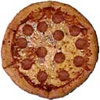Experiment 5: A school survey found that 9 out of 10 students like pizza. If three students are chosen at random with replacement, what is the probability that all three students like pizza?

Probabilities:

 P(student 1 likes pizza) = 9 10P(student 2 likes pizza) = 9 10P(student 3 likes pizza) = 9 10P(student 1 and student 2 and student 3 like pizza) = 9 · 9 · 9 = 729 10 10 10 1000

All of the experiments above involved independent events with a small population (e.g. A 6-sided die, a 2-sided coin, a deck of 52 cards). When a small number of items are selected from a large population without replacement, the probability of each event changes so slightly that the amount of change is negligible. This is illustrated in the following problem.Problem: A nationwide survey found that 72% of people in the United States like pizza. If 3 people are selected at random, what is the probability that all three like pizza?

Solution: Let L represent the event of randomly choosing a person who likes pizza from the U.S.

P(L)  ·  P(L)  ·  P(L)  =  (0.72)(0.72)(0.72) = 0.37 = 37%

In the next lesson, we will address how to handle non-replacement in a small population.

Summary: The probability of two or more independent events occurring in sequence can be found by computing the probability of each event separately, and then multiplying the results together.

### Exercises

Directions: Read each question below. Select your answer by clicking on its button. Feedback to your answer is provided in the RESULTS BOX. If you make a mistake, choose a different button.

 1. Spin a spinner numbered 1 to 7, and toss a coin. What is the probability of getting an odd number on the spinner and a tail on the coin?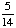None of the above. RESULTS BOX:
 2. A jar contains 6 red balls, 3 green balls, 5 white balls and 7 yellow balls. Two balls are chosen from the jar, with replacement. What is the probability that both balls chosen are green?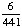None of the above RESULTS BOX:
 3. In Exercise 2, what is the probability of choosing a red and then a yellow ball?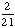All of the above. RESULTS BOX:
 4. Four cards are chosen from a standard deck of 52 playing cards with replacement. What is the probability of choosing 4 hearts in a row?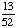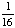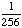None of the above. RESULTS BOX:
 5. A nationwide survey showed that 65% of all children in the United States dislike eating vegetables. If 4 children are chosen at random, what is the probability that all 4 dislike eating vegetables? (Round your answer to the nearest percent.) 18%260%2%None of the above. RESULTS BOX: